# OECD Meat Consumption Data Analysis 5 - scatter plot using R ggplot2::geom_point()Photo by Casey Horner on Unsplash

This post is following of above post.
In this post, let's draw scatter plots using R ggplot2::geom_point.
First of all, let's see correlations about 4 KG_CAPs.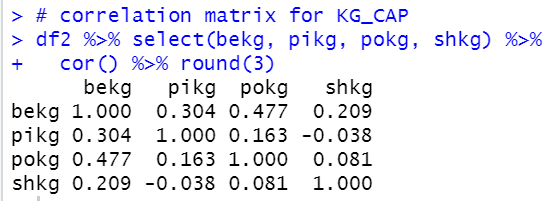bekg: BEEF KG_CAP and pokg: POULTRY KG_CAP are the most strongly correlated pair.

pikg: PIG KG_CAP and shkg: SHEEP KG_CAP are the least weakly correlated pair.

Then, let's draw scatter plots.
First, bekg: BEEF KG_CAP and pikg: PIG KG_CAP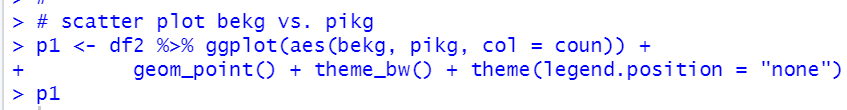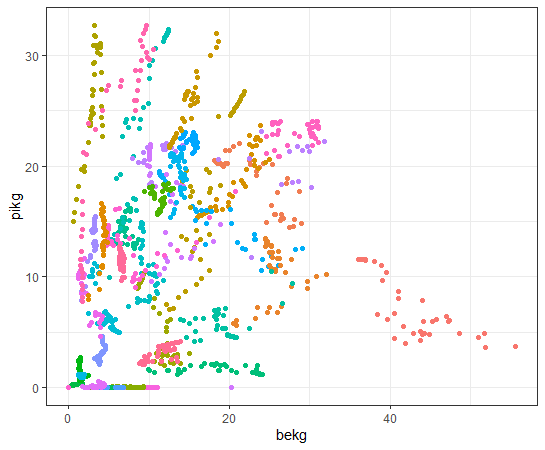We see some countries seems have negative correlation.

Let's see bekg and pokg: POULTRY KG_CAP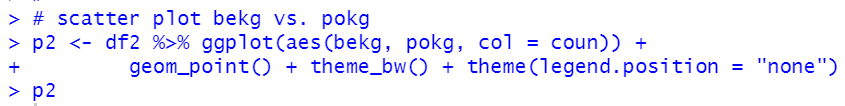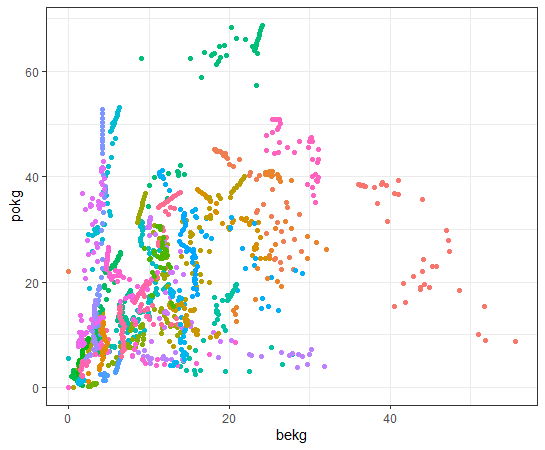How about bekg and shkg: SHEEP KG_CAP?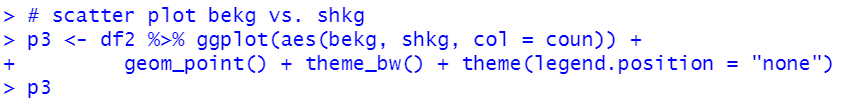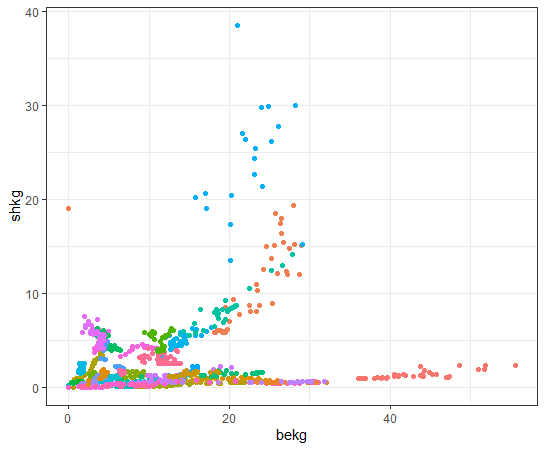Many countries have relatively low value for shkg compared to bekg.

pikg and pokg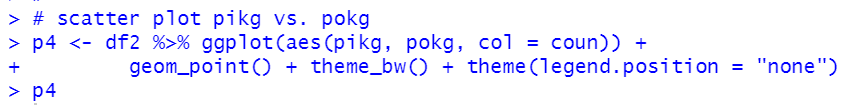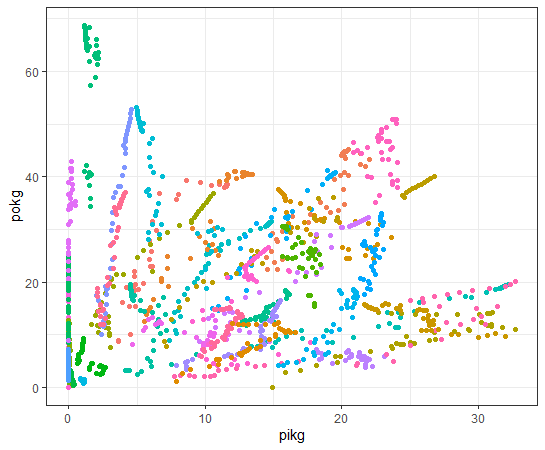pikg and shkg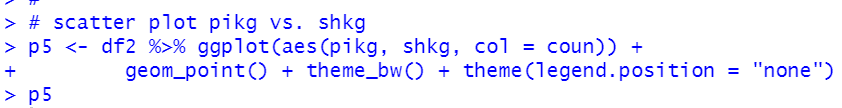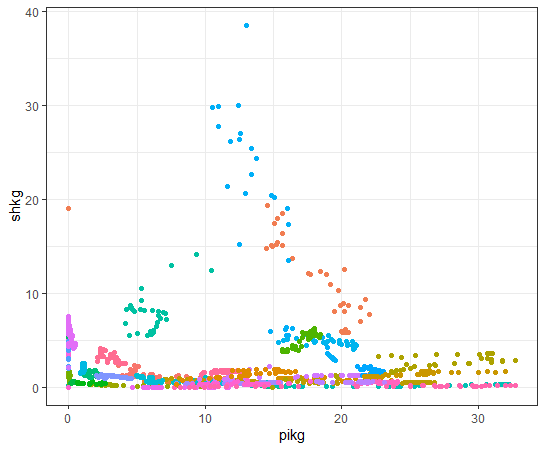Lastly, pokg and shkg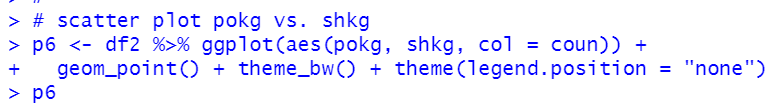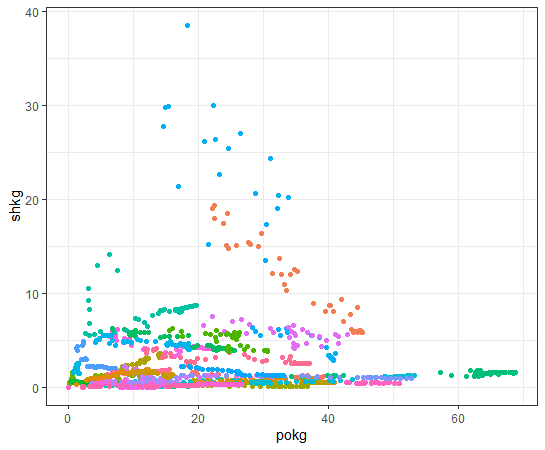Now, we have 6 scatter plot objects, p1 ~ p6.
Let's show it at once. we use gridExtra::grid.arrange() function.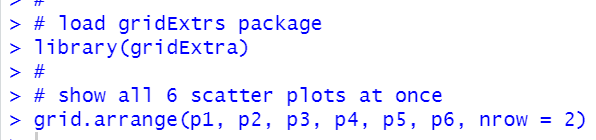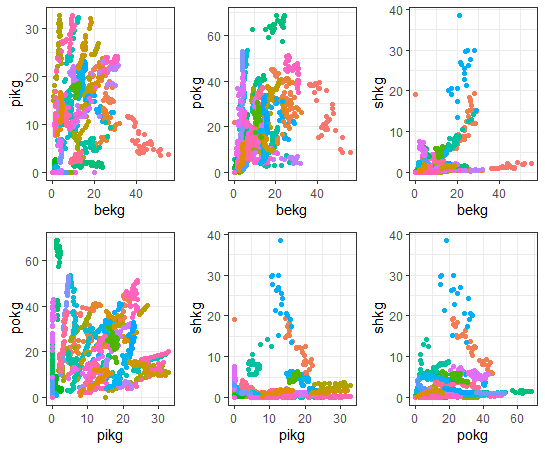That's it. Thank you!

Next post is